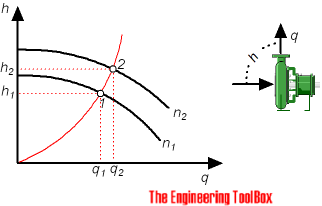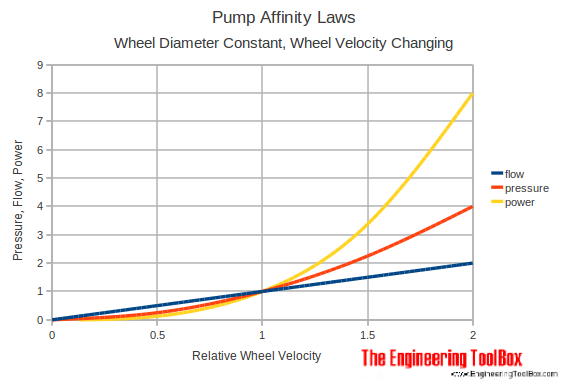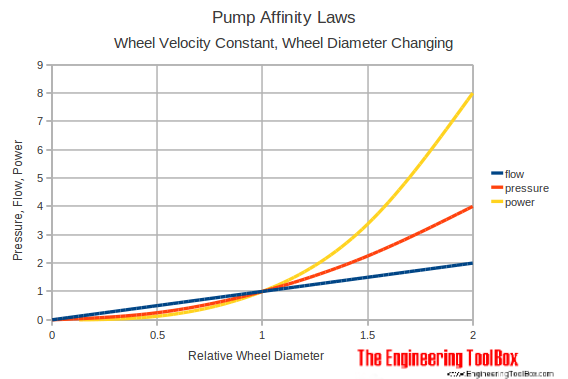Engineering ToolBox - Resources, Tools and Basic Information for Engineering and Design of Technical Applications!

# Pumps - Affinity Laws

## Turbo machines affinity laws can be used to calculate volume capacity, head or power consumption in centrifugal pumps when changing speed or wheel diameters.

The Affinity Laws of centrifugal pumps or fans indicates the influence on volume capacity, head (pressure) and/or power consumption of a pump or fan due to

• change in speed of wheel - revolutions per minute (rpm)
• geometrically similarity - change in impeller diameter

Note that there are two sets of affinity laws:

• affinity laws for a specific centrifugal pump - to approximate head, capacity and power curves for different motor speeds and /or different diameter of impellers
• affinity laws for a family of geometrically similar centrifugal pumps - to approximate head, capacity and power curves for different motor speeds and /or different diameter of impellers

### Pump Affinity Laws for a Specific Centrifugal Pump

#### Volume Capacity

The volume capacity of a centrifugal pump can be expressed like

q1 / q2 = (n1 / n2) (d1 / d2)                                 (1)

where

q = volume flow capacity (m3/s, gpm, cfm, ..)

n = wheel velocity - revolution per minute - (rpm)

d = wheel diameter (m, ft)

The head or pressure of a centrifugal pump can be expressed like

dp1 / dp2 = (n1 / n2)2 (d1 / d2)2                         (2)

where

dp = head or pressure  (m, ft, Pa, psi, ..)

#### Power

The power consumption of a centrifugal pump can be expressed as

P1 / P2 = (n1 / n2)3 (d1 / d2)3                           (3)

where

P = power (W, bhp, ..)

#### Changing Wheel VelocityIf the wheel diameter is constant - change in pump wheel velocity can simplify the affinity laws to

##### Volume Capacity

q1 / q2 = (n1 / n2)                            (1a)

dp1 / dp2 = (n1 / n2)2                             (2a)

##### Power

P1 / P2 = (n1 / n2)3                              (3a)Note! If the speed of a pump is increased with 10%

• the volume flow increases with 10%
• the head increases with 21%
• the power increases with 33 %

If we want to increase the volume flow capacity of an existing system with 10% we have to increase the power supply with 33%.

#### Pump Affinity Laws Calculator - Changing Wheel Velocity

Replace the default values with the actual values. The calculator is generic and can be used with all common units as long as the use is consistent.

q1 - volume capacity - (m3/s, gpm, cfm, ..)

dp1 - head or pressure (m, ft, Pa, psi, ..)

P1 - power (W, bhp)

n1 - initial wheel velocity (rpm)

n2 - final wheel velocity (rpm)

#### Changing the Impeller Diameter

If wheel velocity is constant a change in impeller diameter simplifies the affinity laws to

##### Volume Capacity

q1 / q2 = d1 / d2                           (1b)

dp1 / dp2 = (d1 / d2)2                         (2b)

##### Power

P1 / P2 = (d1 / d2)3                        (3b)#### Pump Affinity Laws Calculator - Changing Wheel Diameter

Replace the default values with the actual values. The calculator is generic and can be used with all common units as long as the use of units is consistent.

q1 - volume capacity - (m3/s, gpm, cfm, ..)

dp1 - head or pressure (m, ft, Pa, psi, ..)

P1 - power (W, bhp)

d1 - initial wheel diameter (m, in, ft  ...)

d2 - final wheel diameter (m, in, ft ..)

#### Example - Pump Affinity Laws - Changing Pump Speed

The pump speed is changed when the impeller size is constant. The initial flow is 100 gpm, the initial head is 100 ft, the initial power is 5 bhp, the initial speed is 1750 rpm and the final speed 3500 rpm.

The final flow capacity can be calculated with (1a):

q2 = q1 n2 / n1

= (100 gpm) (3500 rpm) / (1750 rpm)

= 200 gpm

The final head can be calculated with (2a):

dp2 = dp1 (n2 / n1)2

= (100 ft) ((3500 rpm) / (1750 rpm))2

= 400 ft

The final power consumption can be calculated with (3a):

P2 = P1 (n2 / n1)3

= (5 bhp) ((3500 rpm) / (1750 rpm))3

= 40 bhp

#### Example - Pump Affinity Laws - Changing Impeller Diameter

The diameter of the pump impeller is reduced when the pump speed is constant. The diameter is changed from 8 to 6 inches.

The final flow capacity can be calculated with (1b):

q2 = q1 (d2 / d1)

= (100 gpm) ((¨6 in) / (8 in))

= 75 gpm

The final head can be calculated with (2b):

dp2 = dp1 (d2 / d1)2

= (100 ft) ((6 in) / (8 in))2

= 56.3 ft

The final power consumption can be calculated with (3b):

P2 = P1 (d2 / d1)3

= (5 bhp) ((6 in) / (8 in))3

= 2.1 bhp

### Pump Affinity Laws for a Family of Geometrically Similar Pumps

The volume capacity can be expressed like

q1 / q2 = (n1 / n2)(d1 / d2)3                                        (4)

where

q = volume flow capacity (m3/s, gpm, cfm, ..)

n = wheel velocity - revolution per minute - (rpm)

d = wheel diameter

The head or pressure of a centrifugal pump can be expressed like

dp1 / dp2 = (n1 / n2)2 (d1 / d2)2                                     (5)

where

dp = head or pressure  (m, ft, Pa, psi, ..)

#### Power

The power consumption of a centrifugal pump can be expressed as

P1 / P2 = (n1 / n2)3 (d1 / d2)5                                      (6)

where

P = power (W, bhp, ..)

Note that the affinity laws for fans are not identical with pumps.

## Related Topics

• Fluid Mechanics - The study of fluids - liquids and gases. Involving velocity, pressure, density and temperature as functions of space and time.
• Pumps - Piping systems and pumps - centrifugal pumps, displacement pumps - cavitation, viscosity, head and pressure, power consumption and more.

## Engineering ToolBox - SketchUp Extension - Online 3D modeling!

Add standard and customized parametric components - like flange beams, lumbers, piping, stairs and more - to your Sketchup model with the Engineering ToolBox - SketchUp Extension - enabled for use with the amazing, fun and free SketchUp Make and SketchUp Pro .Add the Engineering ToolBox extension to your SketchUp from the SketchUp Pro Sketchup Extension Warehouse!

Translate

## Privacy

We don't collect information from our users. Only emails and answers are saved in our archive. Cookies are only used in the browser to improve user experience.

Some of our calculators and applications let you save application data to your local computer. These applications will - due to browser restrictions - send data between your browser and our server. We don't save this data.

## Citation

• Engineering ToolBox, (2003). Pumps - Affinity Laws. [online] Available at: https://www.engineeringtoolbox.com/affinity-laws-d_408.html [Accessed Day Mo. Year].

Modify access date.

. .

#### Scientific Online Calculator3 10